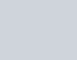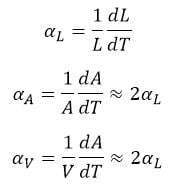Equipment designers must accommodate thermal expansion (CTE)of dissimilar materials, especially when they are subject to large temperature changes.  This problem is often called “CTE mismatch.”  In this blog, we give the fundamentals of thermal expansion calculations used in thermo-mechanical analysis.

These calculations are simple but useful, and easy enough to perform by hand or with a spread sheet. For more complicated shapes, one must use computer modeling.  As an example, we perform a finite element analysis (FEA) in a later blog http://glewengineering.com/thermal-expansion-in-a-glass-and-aluminum-window-part-2/, of a glass and aluminum window and frame to show where the stress is excessive.  This points to the obvious need for a gasket to perform as a thermal interface material, in order to lessen the thermal stress in the glass and avoid fracture.  The need for a gasket is apparent; nearly all of us have seen aluminum window frames with a gasket between the aluminum and the glass.  Nonetheless, it is useful to understand why and how to make that determination.

Single phase materials change in size as their temperature changes, expanding as their temperature increases.  Water expands as it cools and turns to ice, but that is an example of a change in phase, liquid to solid.  Technically, an object’s temperature is just a measure of the energy within it, so increased temperature means increased energy of its atoms.  This leads to greater vibrational magnitude and a shift to larger mean space between atoms.  We commonly see this behavior with gases, since they tend to have the largest change in volume.  A three degree C change in a gas at room temperature results in a 1% expansion.  It’s common knowledge that warm air is less dense than cold air, which can be calculated using the ideal gas law (Equation 1).(1)

Liquids and solids also expand when heated, though to a smaller degree, since their molecules are much more strongly bonded. Hence, the change in size per degree is measured in the parts per million (PPM).  For solids, the potential energy well between atoms is not symmetric, which leads to the average distance between increasing when their energy increases.  The extent that a material expands is governed by that material’s coefficient of thermal expansion (CTE).

## An Introduction to Coefficient of Thermal Expansion for Solids

As one might expect, when gases expand they fill the space they occupy.  Solids, however, have a defined shape and tend to keep that shape as they expand and contract.

We can consider the expansion of any solid object in one, two, or three dimensions, and the CTE can be calculated accordingly, using equations 2 – 4.  These are the change per length, per area, and per volume with each degree of temperature change. These equations all use the same format: the parameter’s rate of change per temperature change, divided by the original value of the parameter.  Books gives tables of CTE values that one uses to predict thermal behavior. Over small temperature changes, one can treat the CTE as a constant.  Over larger temperature changes, one must use different values or a polynomial expression for the CTE, commonly expressed as the Greek letter alhpa “α.”(2) (3) (4)

Where:
α = CTE
L = Length
A = Area
V = Volume

## Engineering for Thermal Expansion

Concrete road beds are very long, so even thought their temperature changes are limited to the relatively small changes in the ambient, the result is that the road expansion can be large.  Thus, engineers use expansion joints, as shown below in Fig. 2, to account for the changes in length of the road beds.  In may be annoying to drive over the expansion joints and experience the bump in the road, but they are necessary.Figure 2: Thermal expansion joint in a bridge
Matt H. Wade. [CC BY-SA 3.0], via Wikimedia Commons